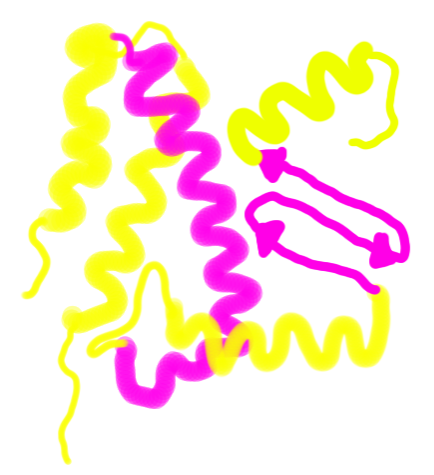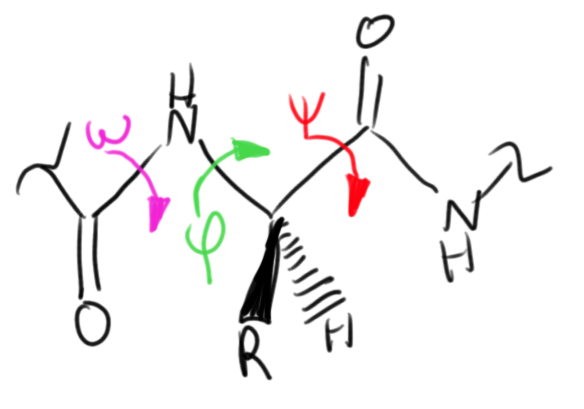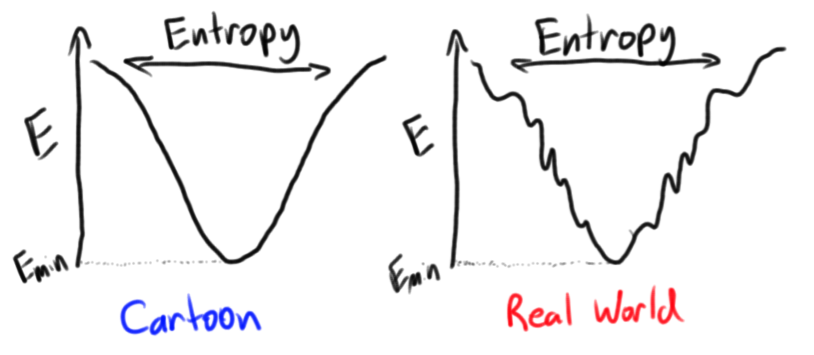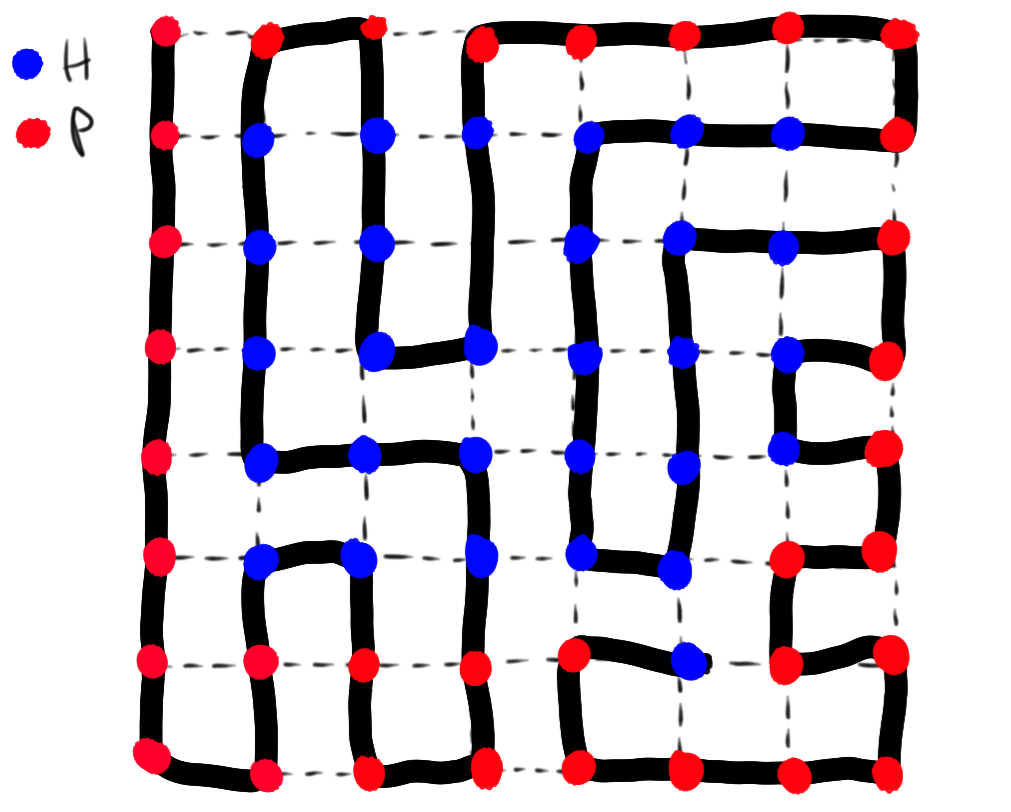# Levinthal's Paradox

too long; didn't read: there's something interesting going on, because proteins should take a trillion years to fold, but they do it in a few milliseconds. maybe evolution hasn't only evolved the folded structure of proteins, but also a greatly accelerated path to get there. or maybe we just have entropy to thank (as usual).

Proteins are generally thought of as the nano-scale machines that do all the hard work in biology. Proteins are the writers, the messengers, and the readers. They serve as soldiers as well as ramparts. Proteins make a big mess, and they do their best to clean it up. The diversity of roles that proteins find themselves in is a perk of their remarkable chemical diversity.

The typical protein is comprised of about 100 amino acid residues assembled in a one-dimensional chain that is folded in three-dimensional space into a "native" folded structure. There exist 20 canonical amino acid residues commonly found in nature (though there are many, more that haven't been used naturally for several geological ages). Given only this sequence diversity, we can draw some boundaries on the sequence space of a typical protein: $r^{N} = 20^{100} \approx 10^{130}$ This is a pretty massive playground of sequence space, being a healthy 50 orders of magnitude greater than the number of protons in the observable universe. Despite the variety of protein folds and functions, this space is very sparsely populated by natural proteins. This is our first clue that a protein is not just a random sequence of amino acids evolution stumbled upon that happens to do a useful purpose : a random point in this sequence space is overwhelmingly likely to be an amino acid sequence that has no stable folded structure or function.

The vanishing likelihood of the blind hand of evolution bumping into one of the lonely functional islands of sequence space is a whole other problem that we shall leave for another day. Instead, we need to consider that each amino acid is a complex molecule of its own, and in order for a protein to be functional, it needs not only the correct sequence of amino acids, but the correct configuration.Even scientists in the field of molecular biology spend much of their time looking at fixed crystal structures of proteins. These structures are solved through crystallographic methods after a large ensemble of proteins have achieved their native conformation. But proteins are not static, and in fact have many conformational degrees of freedom that must be correctly tweaked to end up in the native state. Depending on how you discretize the various configurations of each residue, the configurational space of a protein can be even larger than the sequence space. Each amino acid residue (shown below) has at least three dihedral angles that can rotate independently and must find the one correct orientation to achieve the native state.# The Problem

The process of protein folding is a complex process that starts with an unstructured peptide and ends in a stable globular native fold. In reality, the enthalpic benefits of disulfide, hydrogen and salt bonds interplay with the entropic benefits of hydrophobic collapse in a delicate dance down an energetic funnel towards the native state. But this has far too many moving parts, and we must start much more simply.

Let's look at the folded structure of a protein in a binary manner defined by a sequence of letters $ciiciiciccciciiciiccciicicicci...$ where $c$ represents an amino acid in the "correct" position and conformation (that is, the position and conformation it would be in the native fold), and $i$ represents an amino acid in the "incorrect'' position or conformation (that is, any position or conformation different than the native fold). The native fold is naturally described by a unique sequence $cccccccccccccccccccccccccccccc...$ We can imagine the process of protein folding more simply now. We begin with a random sequence of correct and incorrect amino acids (with the ratio depending on the degeneracy of an incorrect residue relative to a correct one, more on that later), and for each time period $\tau$, each residue can be perturbed and end up in one of the two states. The sequence will meander its way through conformational space until it ends up in the native state.

To make our calculations easier, we can begin by assuming that the two states $c$ and $i$ are equally probable. In this case the probability of the native state appearing given 100 residues is $P=0.5^{100}\approx10^{-30}$ This is a pretty small probability. Given a mole of proteins in random arrangements, we'd still have a negligibly small chance of finding one that was in the native state. Thermodynamically, we can argue that the native state is so-called because it occupies a thermodynamic minima, and that at equilibrium, given an infinite amount of time the population of the native state will be 100%. This tells us that once a protein makes it to the native state, it stays there.

This brings us to the kinetics of the situation. We can tease out the kinetics in a simple way using our understanding of probability. As stated above, the probability of the native state appearing is $P=0.5^{100}$, and thus at any given time, the probability of the native state not appearing is $1-P$. Given the characteristic time step of perturbation $\tau$, the probability that after a given time $t$ the protein remains unfolded is $P_{unfolded}=(1-0.5^{100})^{\frac{t}{\tau}}$ and the probability that after time $t$ the protein will be folded is $P_{folded}=1-(1-0.5^{100})^{\frac{t}{\tau}}$ By setting this probability equal to $0.5$, we can find the $t_{\frac{1}{2}}$ or how long we expect it to take for half the proteins in an ensemble to become folded. We will use this as a characteristic timescale for folding. $t_{\frac{1}{2}}=\tau\frac{log{(0.5)}}{log{(1-0.5^{100}})}$ This expression tells us that the characteristic timescale for folding a 100 residue protein is about $10^{29}\tau$. The observed timescale for folding a typical 100 residue protein is between 100 ms and 1 s. Given the longer time scale, this gives us a perturbation time of $10^{-29}$ seconds, which doesn't even give a photon in vacuum enough time to travel the length of an electron.

Given the perturbation of an amino acid residue requires rearrangement of several covalent bonds, a more reasonable time-scale of perturbation is around one nanosecond ($10^{-9}$ seconds). With this value, the characteristic timescale for folding our 100 residue protein would be about $10^{20}$ seconds, or over three trillion years.

# Solving the Problem with Energy

Keen-eyed readers will see the fallacy we've fallen in to for the sake of simplicity, but it is less obvious whether it has helped us or hurt us. We set the probability of a given residue being perturbed into the correct or incorrect conformation to be equal. In reality, just as for a protein sequence where only one of the innumerable possible conformations is the correct one, there are many incorrect conformations for a given residue, but only one correct one. However, the underlying assumption of the native state is that the correct conformation is energetically favored, and thus is more likely to be occupied than the many incorrect conformations.

To connect these competing interactions to real numbers, we must assign $\alpha$ to be the probability of a given residue of being perturbed into the correct state, with $1-\alpha$ being the probability of falling into the incorrect state. This value represents a statistical bias on our random walk through conformational space. We can state the relative probability of a residue finding the correct conformation $\frac{P(c)}{P(i)}=\frac{\alpha}{1-\alpha}$ To connect this probability to the degeneracy and energetics of the situation, we can turn to statistical mechanics, which tells us that the probability of finding a configuration with energy $E_{a}$ is $P(a)=\frac{g_{a}e^{\frac{-E_{a}}{k_{B}T}}}{Z}$ where $g_{a}$ is the number of configurations of this energy (the degeneracy) and $Z$ is the partition function. Therefore, the relative probability defined above can be simplified to be $\frac{P(c)}{P(i)}=\frac{g_{c}e^{\frac{-E_{c}}{k_{B}T}}}{g_{i}e^{\frac{-E_{i}}{k_{B}T}}}$ We can equate these two relative probabilities and rearrange to interrogate the relative energies and degeneracies by defining $\gamma=\frac{g_{i}}{g_{c}}$ and we end up with $\Delta E=E_{c}-E_{i}=-k_{B}T\log{(\gamma\frac{\alpha}{1-\alpha}})$ The value $\Delta E$ is expected to be negative, representing the energetic benefit of finding the correct conformation. $\gamma$ is likely to be a large positive number, as it is reasonable to assume that there are many more incorrect conformation than correct ones.

Our biased journey through conformational space with a correct bias of $\alpha$ yields a slightly different characteristic timescale of folding $t_{\frac{1}{2}}=\tau\frac{log{(\alpha)}}{log{(1-\alpha^{100}})}$ These two coupled relations allow us to test our cartoon model of protein folding with experimentally relevant values. Let's be generous and let our residues rearrange with a time step of $\tau$=1 ns. We can look at the energetic benefit of finding the correct conformation on a per residue basis in kcal/mol. To get your bearings, the energetic benefit of a hydrogen bond is between 1 and 5 kcal/mol. Table 1 shows enough to get the general trend of these two coupled equations for a protein with $N=100$.

$\begin{array}{c|c|c|c} \alpha & \gamma & \Delta E\text{ (kcal/mol)} & t_{\frac{1}{2}}\text{ (nsec)} \\\hline \ 0.5 & 1 & 0 & >10^{29} \\ \ 0.5 & 10 & 0 & >10^{100} \\ \ 0.9 & 1 & -1.35 & 3900 \\ \ 0.95 & 1 & -1.8 & 8.6 \\ \ 0.9 & 2 & -2.2 & 3900 \\ \ 0.9 & 10 & -3.2 & 3900 \\ \ 0.9 & 100 & -4.6 & 3900 \\ \ 0.9 & 10000 & -7.5 & 3900 \\ \end{array}$

A correct bias of $\alpha=0.9$ allows a 100 residue protein to fold in several microseconds, and a 500 residue protein in about a minute. In the context of our cartoon model, this is approximately the time scale of physiological protein folding, as the longest protein domains observed in nature are about 500 residues, and fold on a timescale of roughly 1 minute.

Though the bias of 0.90 appears to be a seriously loaded dice game, thermodynamic analysis shows us that even with a large relative degeneracy of the incorrect conformation, the energetic benefit required for each correct residue is in a physically possible regime. In a cartoon representation of folding, falling in the vicinity of requiring the formation of a hydrogen bond or two with every correctly positioned residue is surprisingly reasonable.

This model can tell us a bit more about the process of protein folding by looking at what the energetic landscape of the protein folding space would look like. We can extract the shape of the energetic landscape by observing that the energy of a configuration of $N$ residues with $N_{c}$ residues in the correct conformation is $E=N_{c}E_{c}+(N-N_{c})E_{i}$ There exists only one global minimum of this landscape, namely when the protein is in its native state with $N=N_{c}$ and $E_{min}=NE_{c}$. If we move our way up in energy, there are $N$ different states with an energy of $E=(N-1)E_{c}+E_{i}$. This continues with the number of states at each increasing energy according to the number of combinations of $N_{c}$ correct residues in $N$ positions. This is better known as the binomial distribution $n(E)=\frac{N!}{N_{c}!(N-N_{c})!}$ And gives us a corresponding configurational entropy according to its definition of $S=k_{B}T\log{(\frac{N!}{N_{c}!(N-N_{c})!})}$So our landscape starts with an entropy of $0$ (since there is only one native state), and up to a maximum at an equal distribution of correct and incorrect conformations. Thus we have derived the "energy funnel" model of protein folding, in which each stepwise configurational change is modelled to correspond to a decrease in configurational entropy and a decrease in energy. The steepness of this funnel naturally corresponds to our choice of $\alpha$ and the energetic benefit per correct residue. The steepness can also be controlled by constraints on the configurational entropy, which will be investigated in the next section.

# Solving the Problem with Entropy

The solution to the Levinthal paradox outlined above with a cartoon model uses a global bias in the multidimensional energy landscape which pushes the protein towards the native fold. This suggests that the energy funnel arises from evolutionary tuning of the energetically favorable intramolecular interactions. In this view, natural selection is not just responsible for the structure and function of proteins, but also the biologically feasible protein folding times.

As illustrated in the energy funnel model, the "steepness" of the funnel depends not only on the energetic benefit folding, but also the constrained subspace of configurations as the protein gets closer to the native state. That an "entropic force" could serve as a global bias towards protein folding is somewhat contradictory, as thermodynamics tells us that a system will have a tendency towards higher entropy. This collapse of configurational space has been observed experimentally as an ultra-fast first step of protein folding, and has been hypothesized to be caused by hydrophobic forces. As is usually the case, the appearance of entropy decreasing during this spontaneous process is an artefact of not clearly defining the system. The hydrophobic collapse is driven by a relative increase in entropy of the surrounding water molecules in spite of the entropy decrease of the protein chain.

Briefly stated, water molecules enjoy a great deal of translational and rotational freedom in liquid phase by forming an extended hydrogen bond network with a high degree of packing. This network is disrupted by the introduction of a molecule that cannot participate in this network. To minimize the effect on the high entropy network, water molecules wrap tangentially around the hydrophobic surface and participate in hydrogen bonds with one another to form a highly-ordered water cage. This represents a very large entropic penalty, and thus the system will tend towards a hydrophobic collapse, in which the hydrophobic residues of a protein coalesce into a droplet with minimal surface area.

To minimize the water cage, the system is willing to pay the entropic penalty that comes with spatially constrained hydrophobic core of protein residues. This is the global bias that drastically constrains the configuration space (number of unique protein conformations) of proteins while folding. $n(\text{folds})=\gamma^{N}$ where N is the length of the protein chain and $\gamma$ is the configurational degeneracy of each residue (a relatively high number as outlined in the previous section). It is this relation that first confused Levinthal in his 1969 paper, where he discretized the possible configurations of each residue into $\gamma=10$ possible states, but still ended up with a configurational space far too vast to sample in a reasonable amount of time. But the hydrophobic collapse forces the protein into a well-packed globular structure, and it becomes reasonable to represent the chain as a Hamiltonian path on a lattice. This is a model reminiscent of a game of Snake: a self-avoiding walk passing through each point in a lattice once and only once.The number of unique Hamiltonian paths $\mathcal{N}_{H}$ given $N$ residues has a mean-field limit that can be analytically determined $\mathcal{N}_{H} = \gamma_{H}^{N}$ $\log{\gamma_{H}}=\lim_{N\to\infty} \frac{1}{N}\mathcal{N}_{H}$ $\lim_{N\to\infty} \gamma_{H}=\frac{q}{e}$ In the case of a course-grained cubic lattice with 6 interfaces ($q$) on each cubic "residue", the per-residue degeneracy can be significantly reduced to $\gamma_{H}=\frac{6}{e}\approx2.2$ Though we've gotten rid of several dozen orders of magnitude compared to Levinthal's original unbiased folding, this well-packed model of the protein completely ignores the molecular basis of hydrophobic collapse: hydrophobic residues maximize contact with other hydrophobic residues and minimize contact with polar residues. We must build another simple binary model of protein sequences to better understand how to take this into account: $HPPPHHHPPPHHHHPPHHHPPHHPP$ where $H$ represents a hydrophobic residue (alanine, leucine, isoleucine, phenylalanine, etc.) and $P$ represents a polar residue (serine, glutamine, arginine, etc.). This approach is known as the HP model, and is also found as a cartoon model to understand sequence alignment in computational biology. We can further constrain our configurational space by limiting our analysis to those Hamiltonian paths on the lattice that have optimal contact between $H$ residues and minimizes contact between $H$ and $P$ residues. These circuitous Hamiltonian paths are the game of Snake from hell, and finding them is much easier said than done. However a recent startling analytical result has shown that this model can further decrease the per residue degeneracy to $\gamma_{HP}=\frac{3}{e}\approx 1.1$ assuming equal amounts of hydrophobic and polar residues. Though there is no getting around the exponential dependence of configurational space on number of residues, these types of rationally designed models can set limits on the base of the exponent. The HP model is sufficient to explain the physiologically feasible folding times (microseconds to seconds) of proteins up to 200 residues.

Table 2 shows the effect on protein folding timescales of this various models for a protein with length $N=100$: $\begin{array}{c|c|c} \text{Model} & \gamma & t_{\frac{1}{2}} \text{ (nsec)} \\\hline \text{Continuous} & \infty & \infty \\ \text{Levinthal} & 10 & 10^{100} \\ \text{Hamiltonian} & \approx2.2 & 10^{34} \\ \text{HP} & \approx1.1 & 19000 \\ \end{array}$

# Caveats

Note that this document is still called Levinthal's Paradox, and not Levinthal's Obvious Answer. The two approaches to understanding the global bias towards the native state that is necessitated by the paradox do not have all the answers. Both give reasonable approximations to experimentally observed dynamics, but there are some glaring contradictions.

Careful calorimetry of proteins during folding have observed far less than the 1-10 kcal/mol per residue energetic benefit called for by the first solution. Most proteins average $\approx0.2$ kcal/mol per residue. This discrepency may be explained by the additional entropic driving force of the hydrophobic collapse, but the course-grained nature of that model has yet to be computationally observed in a more explicit molecular dynamics simulation of protein folding.

There is still much more to be discovered.

# Further Reading

Levinthal, C. How to Fold Graciously. In Mossbauer Spectroscopy in Biological Systems, Proceedings of a Meeting held at Allerton House, Monticello, Illinois; Debrunner, P., Tsibris, J. C. M., Munck, E., Eds.;University of Illinois Press: Urbana, 1969.

Milo M. Lin and Ahmed H. Zewail Hydrophobic forces and the length limit of foldable protein domains, PNAS 2012 109 (25) 9851-9856

Zwanzig R, Szabo A, Bagchi B. Levinthal’s paradox., PNAS 1992;89(1):20-22.Note by Blake Farrow
3 years, 11 months ago

This discussion board is a place to discuss our Daily Challenges and the math and science related to those challenges. Explanations are more than just a solution — they should explain the steps and thinking strategies that you used to obtain the solution. Comments should further the discussion of math and science.

When posting on Brilliant:

• Use the emojis to react to an explanation, whether you're congratulating a job well done , or just really confused .
• Ask specific questions about the challenge or the steps in somebody's explanation. Well-posed questions can add a lot to the discussion, but posting "I don't understand!" doesn't help anyone.
• Try to contribute something new to the discussion, whether it is an extension, generalization or other idea related to the challenge.
• Stay on topic — we're all here to learn more about math and science, not to hear about your favorite get-rich-quick scheme or current world events.

MarkdownAppears as
*italics* or _italics_ italics
**bold** or __bold__ bold
- bulleted- list
• bulleted
• list
1. numbered2. list
1. numbered
2. list
Note: you must add a full line of space before and after lists for them to show up correctly
paragraph 1paragraph 2

paragraph 1

paragraph 2

[example link](https://brilliant.org)example link
> This is a quote
This is a quote
    # I indented these lines
# 4 spaces, and now they show
# up as a code block.

print "hello world"
# I indented these lines
# 4 spaces, and now they show
# up as a code block.

print "hello world"
MathAppears as
Remember to wrap math in $$ ... $$ or $ ... $ to ensure proper formatting.
2 \times 3 $2 \times 3$
2^{34} $2^{34}$
a_{i-1} $a_{i-1}$
\frac{2}{3} $\frac{2}{3}$
\sqrt{2} $\sqrt{2}$
\sum_{i=1}^3 $\sum_{i=1}^3$
\sin \theta $\sin \theta$
\boxed{123} $\boxed{123}$

## Comments

There are no comments in this discussion.

×

Problem Loading...

Note Loading...

Set Loading...